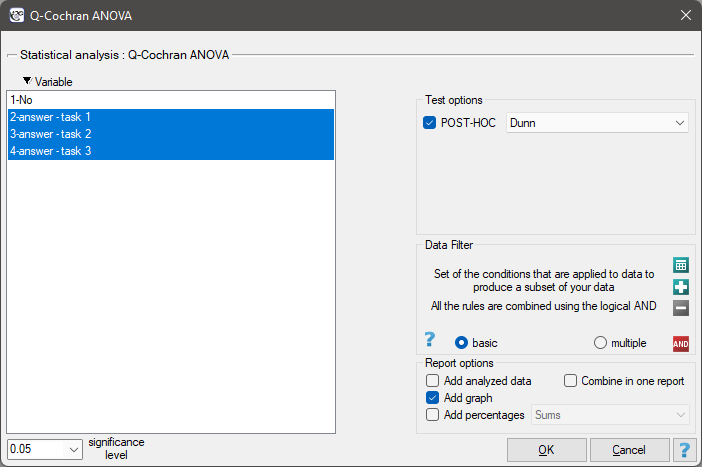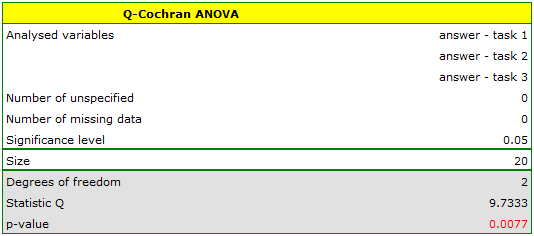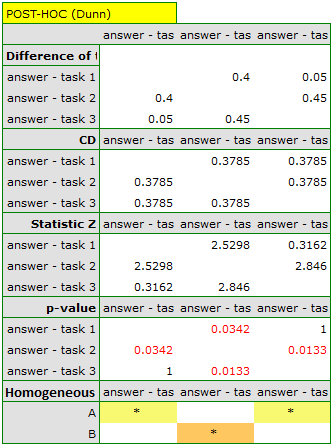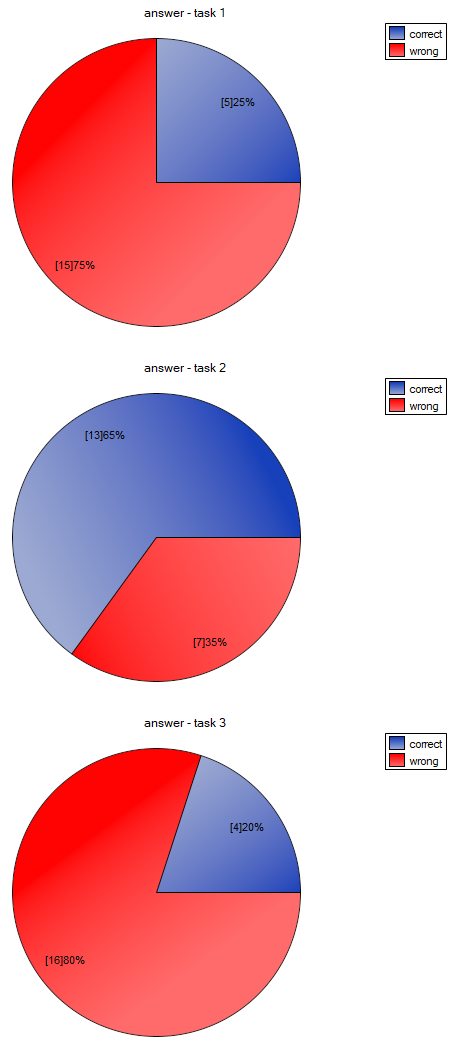#PQStat - Baza Wiedzy

### Pasek boczny

en:statpqpl:porown3grpl:nparpl:anova_qcochrpl

### The Q-Cochran ANOVA

The Q-Cochran analysis of variance, based on the Q-Cochran test, is described by Cochran (1950)1). This test is an extended McNemar test fordependent groups. It is used in hypothesis verification about symmetry between several measurementsfor thefeature. The analysed feature can have only 2 values - for the analysis, there are ascribed to them the numbers: 1 and 0.

Basic assumptions:

• measurement on a nominal scale (dichotomous variables – it means the variables of two categories),

Hypotheses:where:

„incompatible” observed frequencies – the observed frequencies calculated when the value of the analysed feature is different in several measurements.

The test statistic is defined by:where:,,,– the value of-th measurement for-th object (so 0 or 1).

This statistic asymptotically (for large sample size) has the Chi-square distribution with a number of degrees of freedom calculated using the formula:.

The p-value, designated on the basis of the test statistic, is compared with the significance level:The POST-HOC tests

Introduction to the contrasts and the POST-HOC tests was performed in the unit, which relates to the one-way analysis of variance.

The Dunn test

For simple comparisons (frequency in particular measurements is always the same).

Hypotheses:

Example - simple comparisons (for the difference in proportion in a one chosen pair of measurements):• [i] The value of critical difference is calculated by using the following formula:where:- is the critical value (statistic) of the normal distribution for a given significance levelcorrected on the number of possible simple comparisons.

</WRAP

• [ii] The test statistic is defined by:where:– the proportion-th measurement,

The test statistic asymptotically (for large sample size) has the normal distribution, and the p-value is corrected on the number of possible simple comparisons.

The settings window with the Cochran Q ANOVA can be opened in Statistics menu→ NonParametric testsCochran Q ANOVA or in ''Wizard''.Note

This test can be calculated only on the basis of raw data.

EXAMPLE(test.pqs file)

We want to compare the difficulty of 3 test questions. To do this, we select a sample of 20 people from the analysed population. Every person from the sample answers 3 test questions. Next, we check the correctness of answers (an answer can be correct or wrong). In the table, there are following scores:Hypotheses:Comparing the p value p=0.0077 with the significance levelwe conclude that individual test questions have different difficulty levels. We resume the analysis to perform POST-HOC test by clicking, and in the test option window, we select POST-HOC Dunn.The carried out POST-HOC analysis indicates that there are differences between the 2-nd and 1-st question and between questions 2-nd and 3-th. The difference is because the second question is easier than the first and the third ones (the number of correct answers the first question is higher).

1)
Cochran W.G. (1950), The comparison ofpercentages in matched samples. Biometrika, 37, 256-266
en/statpqpl/porown3grpl/nparpl/anova_qcochrpl.txt · ostatnio zmienione: 2022/02/13 17:35 przez admin

### Narzędzia strony